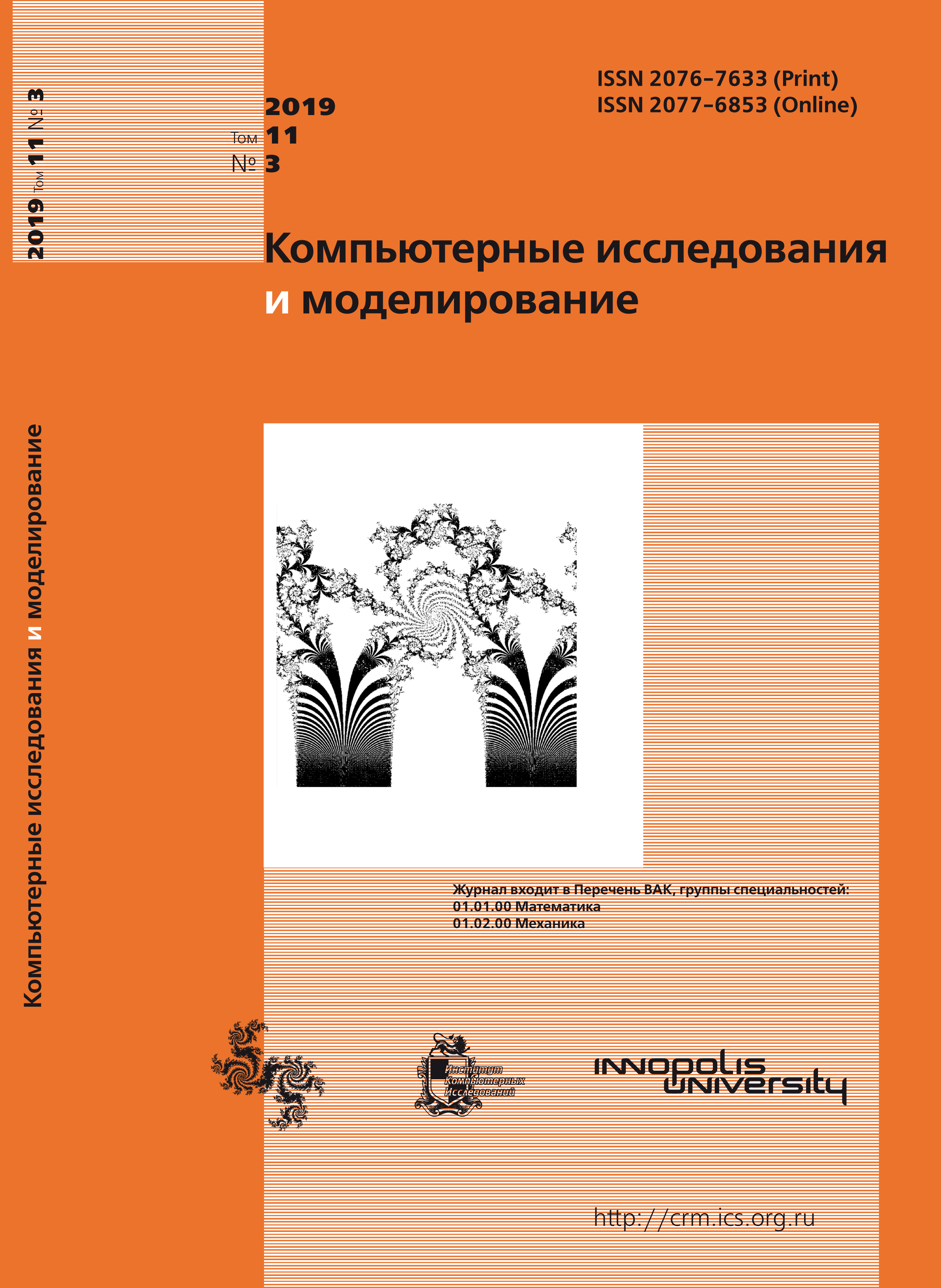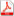Issue 3, 2019 Vol. 11

# All issues

Solving of the Exner equation for morphologically complex bedpdf (308K)  / List of references

The Exner equation in conjunction phenomenological sediment transport models is widely used for mathematical modeling non-cohesive river bed. This approach allows to obtain an accurate solution without any difficulty if one models evolution of simple shape bed. However if one models evolution of complex shape bed with unstable soil the numerical instability occurs in some cases. It is difficult to detach this numerical instability from the natural physical instability of bed.

This paper analyses the causes of numerical instability occurring while modeling evolution of complex shape bed by using the Exner equation and phenomenological sediment rate models. The paper shows that two kinds of indeterminateness may occur while solving numerically the Exner equation closed by phenomenological model of sediment transport. The first indeterminateness occurs in the bed area where sediment transport is transit and bed is not changed. The second indeterminateness occurs at the extreme point of bed profile when the sediment rate varies and the bed remains the same. Authors performed the closure of the Exner equation by the analytical sediment transport model, which allowed to transform the Exner equation to parabolic type equation. Analysis of the obtained equation showed that it’s numerical solving does not lead to occurring of the indeterminateness mentioned above. Parabolic form of the transformed Exner equation allows to apply the effective and stable implicit central difference scheme for this equation solving.

The model problem of bed evolution in presence of periodic distribution of the bed shear stress is carried out. The authors used the explicit central difference scheme with and without filtration method application and implicit central difference scheme for numerical solution of the problem. It is shown that the explicit central difference scheme is unstable in the area of the bed profile extremum. Using the filtration method resulted to increased dissipation of the solution. The solution obtained by using the implicit central difference scheme corresponds to the distribution law of bed shear stress and is stable throughout the calculation area.

Keywords: mathematical modeling, numerical instability, Exner equation, river bed, sediment transport, analytical model
Citation in English: Potapov I.I., Snigur K.S. Solving of the Exner equation for morphologically complex bed // Computer Research and Modeling, 2019, vol. 11, no. 3, pp. 449-461
Citation in English: Potapov I.I., Snigur K.S. Solving of the Exner equation for morphologically complex bed // Computer Research and Modeling, 2019, vol. 11, no. 3, pp. 449-461
DOI: 10.20537/2076-7633-2019-11-3-449-461
Views (last year): 10.

Full-text version of the journal is also available on the web site of the scientific electronic library eLIBRARY.RU

The journal is included in the Russian Science Citation Index

The journal is included in the List of Russian peer-reviewed journals publishing the main research results of PhD and doctoral dissertations.

International Interdisciplinary Conference "Mathematics. Computing. Education"

The journal is included in the RSCI

Indexed in Scopus